# How to Find Perimeter

## How to Find Perimeter of Triangles, Perimeter of Rectangles, and Other Polygons With Examples And Interactive Exercises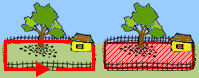The perimeter of a polygon is the distance around the outside of the polygon. A polygon is 2-dimensional; however, perimeter is 1-dimensional and is measured in linear units. To help us make this distinction, look at our picture of a rectangular backyard. The yard is 2-dimensional: it has a length and a width. The amount of fence needed to enclose the backyard (perimeter) is 1-dimensional. The perimeter of this yard is the distance around the outside of the yard, indicated by the red arrow; It is measured in linear units such as feet or meters.

To find the perimeter of a polygon, take the sum of the length of each side. The polygons below are much smaller than a fenced-in yard. Thus, we use smaller units in our examples, such as centimeters and inches.Example 1: Find the perimeter of a triangle with sides measuring 5 centimeters, 9 centimeters and 11 centimeters.

Solution: P = 5 cm + 9 cm + 11 cm = 25 cm

###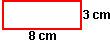Example 2: A rectangle has a length of 8 centimeters and a width of 3 centimeters. Find the perimeter.

Solution 1: P = 8 cm + 8cm + 3 cm + 3 cm = 22 cm

Solution 2: P = 2(8 cm) + 2(3 cm) = 16 cm + 6 cm = 22 cm

### In Example 2, the second solution is more commonly used. In fact, in mathematics, we commonly use the following formula for perimeter of a rectangle: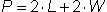where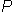is the perimeter,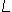is the length and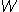is the width.

In the next few examples, we will find the perimeter of other polygons.

###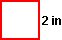Example 3: Find the perimeter of a square with each side measuring 2 inches.

Solution:= 2 in + 2 in + 2 in + 2 in = 8 in

###Example 4: Find the perimeter of an equilateral triangle with each side measuring 4 centimeters.

Solution:= 4 cm + 4 cm + 4 cm = 12 cm

###Example 3: Find the perimeter of a square with each side measuring 2 inches.

Solution: This regular polygon has 4 sides, each with a length of 2 inches. Thus we get:= 4(2 in) = 8 in

###Example 4: Find the perimeter of an equilateral triangle with each side measuring 4 centimeters.

Solution: This regular polygon has 3 sides, each with a length of 4 centimeters. Thus we get:= 3(4 cm) = 12 cm

###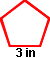Example 5: Find the perimeter of a regular pentagon with each side measuring 3 inches.

Solution:= 5(3 in) = 15 in

###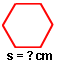Example 6: The perimeter of a regular hexagon is 18 centimeters. How long is one side?

Solution:= 18 cm

Let S represent the length of one side. A regular hexagon has 6 sides, so we can divide the perimeter by 6 to get the length of one side (S).

S = 18 cm ÷ 6

S = 3 cm

### Directions: Read each question below. Click once in an ANSWER BOX and type in your answer; then click ENTER. Your answers should be given as whole numbers greater than zero. After you click ENTER, a message will appear in the RESULTS BOX to indicate whether your answer is correct or incorrect. To start over, click CLEAR.

 1 Find the perimeter of a triangle with sides measuring 10 inches, 14 inches and 15 inches.  ANSWER BOX:=  in  RESULTS BOX:
 2 A rectangle has a length of 12 centimeters and a width of 4 centimeters. Find the perimeter. ANSWER BOX:=  cm  RESULTS BOX:
 3 Find the perimeter of a regular hexagon with each side measuring 8 meters. ANSWER BOX:=  m  RESULTS BOX:
 4 The perimeter of a square is 20 feet. How long is each side? ANSWER BOX:=  ft  RESULTS BOX:
 5 The perimeter of a regular pentagon is 100 centimeters. How long is each side? ANSWER BOX:=  cm  RESULTS BOX: